# Welcome to the SynBioSoc's first image analysis workshop!¶

Let's start by importing everything we're going to need.

In :
import matplotlib.pyplot as plt
from matplotlib.pyplot import imshow
import numpy as np
from PIL import Image
import requests
from scipy.ndimage.morphology import grey_opening, grey_closing, grey_dilation, grey_erosion, binary_opening, binary_closing, binary_dilation, binary_erosion
from skimage.filters import (threshold_otsu, threshold_niblack,
threshold_sauvola)


1. We're going to load an image using the pillow library in Python.
2. We have the image hosted on the society's webspace, so we can use the requests library to immediately download it.
In :
im = Image.open(requests.get("http://cusbs.soc.srcf.net/datasets/image_analysis/mother_machine_example.tif", stream=True).raw)


# Images are arrays¶

Images are just an array (or matrix) of numbers. Each element in the array corresponds to a pixel, and the number in that element corresponds to the intensity of that pixel.

## Images come in a few varieties:¶

• Images can be in colour, or greyscale. A colour image is actually 3 greyscale images, one for each colour channel (most commonly RGB).
• In this talk we will focus only on greyscale images, because they are simpler to work with, and are the output of most microscopes.

We can convert this image into numpy array by using the following comand:

In :
im = np.asarray(im)


Working with numpy arrays is easy. Let's take a look at the array of this image:

In :
im

Out:
array([[162, 193, 151, ..., 180, 222, 181],
[184, 186, 171, ..., 151, 177, 246],
[188, 170, 208, ..., 213, 186, 230],
...,
[156, 194, 168, ..., 200, 178, 178],
[206, 187, 216, ..., 201, 213, 185],
[171, 216, 211, ..., 182, 190, 195]], dtype=uint16)
• This image is greyscale. This means that the numpy array is 2-Dimensional.
• If you were to load in an RGB image, you would actually see an NxMx3 matrix. If you loaded in a .png with an alpha channel (transparency) you would see NxMx4
• Above, we can see a subset of the image elements.
• We can also see that the data type of the pixels in this image are unint16. This means we are working with a 16-bit image.
• This array is also only 2 dimensional, meaning we have one colour channel. We can therefore consider this image to be greyscale.
• A 16-bit greyscale image can have up to $65,536$ (which is $2^{16}$) unique pixel intensities.

# Slicing arrays¶

Arrays use row-column indexing. To slice the top row (row 0) of this image we can write:

In :
im[0,:]

Out:
array([162, 193, 151, ..., 180, 222, 181], dtype=uint16)

To slice the first column:

In :
im[:,0]

Out:
array([162, 184, 188, ..., 156, 206, 171], dtype=uint16)

We can also slice multiple rows and columns.

For example, we can take the first 10 rows:

In :
im[0:10,:]

Out:
array([[162, 193, 151, ..., 180, 222, 181],
[184, 186, 171, ..., 151, 177, 246],
[188, 170, 208, ..., 213, 186, 230],
...,
[186, 192, 149, ..., 168, 179, 168],
[172, 179, 213, ..., 164, 169, 178],
[198, 178, 192, ..., 205, 163, 216]], dtype=uint16)

Or the 31st-434th column:

In :
im[:,31:434]

Out:
array([[156, 205, 160, ..., 203, 200, 193],
[188, 182, 176, ..., 185, 202, 215],
[188, 172, 207, ..., 220, 187, 199],
...,
[201, 190, 152, ..., 240, 227, 210],
[199, 201, 199, ..., 192, 194, 199],
[194, 199, 157, ..., 243, 206, 198]], dtype=uint16)

# Displaying images using matplotlib¶

We can call imshow from the matplotlib library to take a look at our array!

In :
plt.figure(figsize=(10,10))
imshow(im)
plt.show()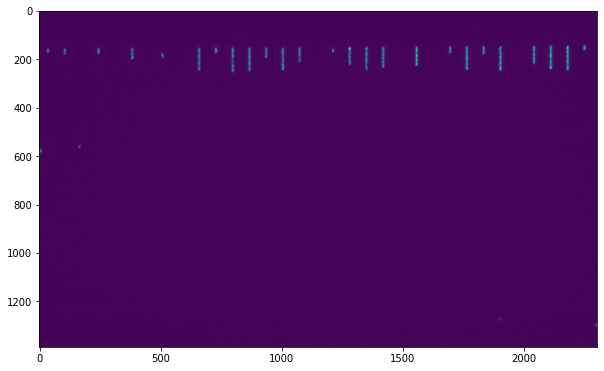It's not very clear what we're looking at. Let's take a closer look.

• These cells are in a mother machine, and so are lined up in "trenches".
• We'll take a closer look at them by slicing.
• Let's slice out what looks to be the interesting part of the image.
• It looks like the cells are in the entire y-axis (all columns) but only from row ~130 to ~270
In :
all_cells = im[130:270,:]

In :
plt.figure(figsize=(20,20))
imshow(all_cells)
plt.show()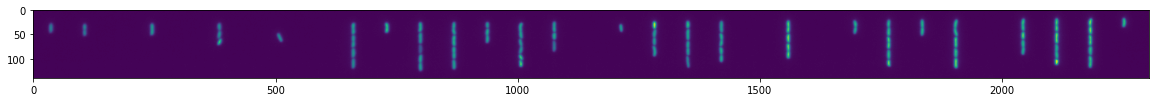Let's focus in on a single trench of cells and see what we can do! (I've already figured out what the indexes should be for a group of cells).

In :
cells = all_cells[:,850:890]

In :
plt.figure(figsize=(5,5))
imshow(cells)

Out:
<matplotlib.image.AxesImage at 0x7f14275f15b0>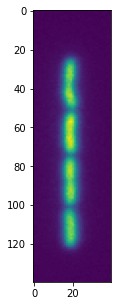# Greyscale morphological operators¶

• We have imported 4 morphological operators which work on greyscale images. These are:
• grey_opening
• grey_closing
• grey_dilation
• grey_erosion

We won't go into the detail of how these operators work, but we can get an intuition for what they do by playing around with them, and one of the parameters which these functions take, which is size.

Here is an example where we've transformed the original image using grey_openinng with a volume element of size 5x5.

In :
cells_grey_erosion = grey_erosion(cells,size=(5,5))
cells_grey_dilation = grey_dilation(cells,size=(5,5))
cells_grey_opening = grey_opening(cells,size=(5,5))
cells_grey_closing = grey_closing(cells,size=(5,5))
fig, axs = plt.subplots(1,5)
axs.imshow(cells)
axs.set_title("Original")
axs.imshow(cells_grey_erosion)
axs.set_title("Erosion")
axs.imshow(cells_grey_dilation)
axs.set_title("Dilation")
axs.imshow(cells_grey_opening)
axs.set_title("Opening")
axs.imshow(cells_grey_closing)
axs.set_title("Closing")
for ax in axs:
ax.axis("off")
plt.show()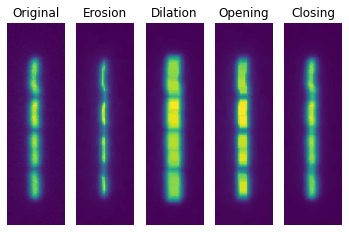# Thresholding¶

We will consider 3 different thresholding methods here. One is the famous Otsu thresholding method, which is a global thresholding method. This finds critical intensity and thresholds the image by setting all intensities lower than the critical to be 0, and all above the critical to be 1.

We also look at Niblack and Sauvola thresholding which operate on local areas of the image, using a window of a certain size to locally threshold regions of the image. These tend to perform better on images with large differences in intensity and contrast.

In :
def try_all_threshold(image, window_size, k):
binary_otsu = image > threshold_otsu(image)

thresh_niblack = threshold_niblack(image, window_size=window_size, k=k)
thresh_sauvola = threshold_sauvola(image, window_size=window_size)

binary_niblack = image > thresh_niblack
binary_sauvola = image > thresh_sauvola

fig, axs = plt.subplots(1,4)
axs.imshow(image)
axs.set_title("Original")
axs.imshow(binary_otsu)
axs.set_title("Otsu")
axs.imshow(binary_niblack)
axs.set_title("Niblack")
axs.imshow(binary_sauvola)
axs.set_title("Sauvola")
for ax in axs:
ax.axis("off")
plt.show()
return binary_otsu, binary_niblack, binary_sauvola

In :
thresholded = try_all_threshold(cells, 15, 0.2)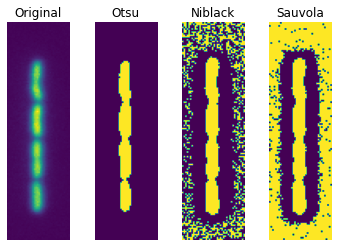We can see that these thresholding techniques are not performing perfectly on the first attempt. You can try playing wound with with window_size and k parameters which will affect the local thresholding methods. But we can perform some other tricks to clean things up:

Let's first remove all the junk which is cluttering our Niblack and Sauvola thresholds. We can do this by removing uninteresting background pixels by setting a manual threshold to turn all pixels below a certain intensity to 0.

A good starting point for what the background might be is to actually check the critical intensity from Otsu's method.

In :
threshold_otsu(cells) #
cells_nobg = cells * (cells > threshold_otsu(cells)*1.5)
thresholded = try_all_threshold(cells_nobg, 15, 0.2)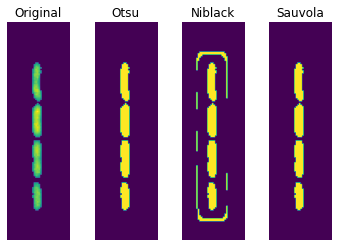All 3 thresholding methods look pretty good now! We can actually clean up the Niblack result with binary morphological operators.

We can try doing opening again:

In :
niblack_opened = binary_opening(thresholded)
imshow(niblack_opened)
plt.show()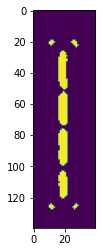In fact, greyscale morphological operators give alternative methods of fixing up our images. Let's go back to when we had no background subtraction:

In :
thresholded = try_all_threshold(cells, 15, 0.2)Let's try some binary operators on Niblack

In :
niblack_opened = binary_opening(thresholded)
imshow(niblack_opened)
plt.show()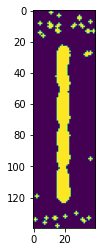Some weird spots left over from the binary opening. Let's clear them up with an erosion and then call opening again to clean up the gaps between cells:

In :
niblack_eroded = binary_erosion(thresholded)
niblack_eroded_opened = binary_opening(niblack_eroded, structure=np.ones((1,5)))
imshow(niblack_eroded_opened)

Out:
<matplotlib.image.AxesImage at 0x7f1425840a60>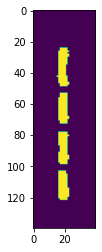In :
thresholded = try_all_threshold(all_cells, 15, 0.2)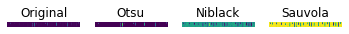In :
plt.figure(figsize=(30,30))
niblack_eroded = binary_erosion(thresholded)
niblack_eroded_opened = binary_opening(niblack_eroded, structure=np.ones((1,5)))
imshow(niblack_eroded_opened)
plt.show()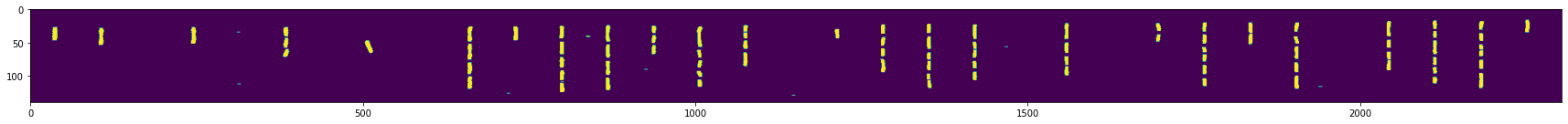In [ ]: